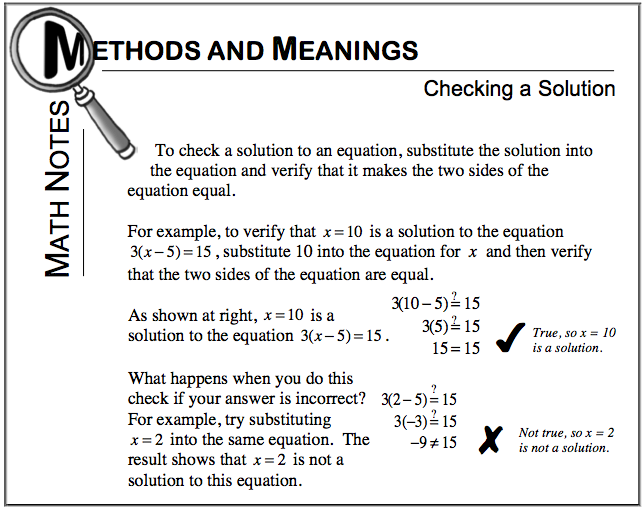### Home > CC2 > Chapter Ch6 > Lesson 6.2.3 > Problem6-81

6-81.

Solve each equation below for $x$. Check your final answer. Homework Help ✎

1. $4x=6x-14$

Subtract $6x$ from both sides.

$\array{\qquad \ \ \ 4x=6x-14\\ -6x\ \ -6x}\\ \quad \ \ \ −2x = −14$

Divide each side by $−2$.

${\frac{-2\textit{x}}{-2}=\frac{-14}{-2}}$

$x=7$

1. $3x+5=50$

Try using the same method presented in part (a).